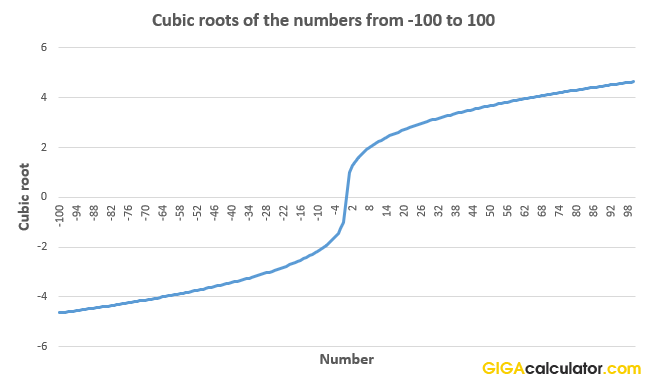# Cube Root Calculator

Use this cube root calculator to easily calculate the cube root of a given number.

Share calculator:

Embed this tool:
get code

## What is a cube root?

The cube root of a number answers the question "what number can I multiply by itself twice to get this number?". It is the reverse of the exponentiation operation with an exponent of 3, so if r3 = x, then we say that "r is the cube root of x". Finding the root of a number has a special notation called the radical symbol: √ - which is used as it is when it comes to square roots, but gets an index number indicating the root - 3 in the case of the cube root, so it looks like so: ∛. Usually the radical spans over the entire equation for which the root is to be found. It is called a "cube" root since multiplying a number by itself twice is how one finds the volume of a cube.

Unlike the square root, there is only one unique real number root as a result from applying the cube root function for a given number and it carries the sign of the number. For example, the ∛8 = 2, while ∛-8 = -2, since 2 x 2 x 2 = 8 and -2 x -2 x -2 = -8. The real number cube root is the Principal cube root, but each real number cube root (zero excluded) also has a pair of complex conjugate roots. For example, the other cube roots of 8 are -1 + √3i and -1 - √3i. Our cube root calculator will only output the principal root. See the table of common roots below for more examples.

When interpreting the output of the calculator it might help to know that in geometrical terms, the cube root function maps the volume of a cube onto its side length.

## Properties and practical application of the cube root

Cubic roots are useful when one needs to divide an angle in three, that is - to find an angle whose measure is one third of a given angle. This operation is called angle trisection. Cube roots are used when you need to find the edge of a cube whose volume is twice that of a cube with a given edge.Plotting the results from the cube root function, as calculated using this calculator, on a graph reveals that it has the shape of half a parabola.

## Commonly used cube roots

Table of commonly encountered cube roots:

Commonly used cube roots
Cubic Root ofRoot
∛1 1
∛8 2
∛27 3
∛64 4
∛125 5
∛216 6
∛1,000 10
∛1,000,000 100
∛1,000,000,000 1000

The calculations were performed using this cube root calculator.

## Does the calculator support fractions?

Yes, simply enter the fraction as a decimal floating point number and you will get the corresponding cube root. For example, to compute the cube root of 1/2 simply enter 0.5 in the input field and you will get 0.7937 as ouput. If you are having trouble converting a fraction to a decimal number, you will find our fraction to decimal converter handy.

#### Cite this calculator & page

If you'd like to cite this online calculator resource and information as provided on the page, you can use the following citation:
Georgiev G.Z., "Cube Root Calculator", [online] Available at: https://www.gigacalculator.com/calculators/cube-root-calculator.php URL [Accessed Date: 27 Mar, 2023].